## A note on the hopes for Fully Homomorphic Signatures

This is taken from my Master Thesis on Homomorphic Signatures over Lattices.

## What are homomorphic signatures

Imagine that Alice owns a large data set, over which she would like to perform some computation. In a homomorphic signature scheme, Alice signs the data set with her secret key and uploads the signed data to an untrusted server. The server then performs the computation modeled by the function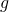to obtain the result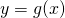over the signed data.

Alongside the result, the server also computes a signature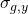certifying thatis the correct result for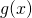. The signature should be short – at any rate, it must be independent of the size of. Using Alice’s public verification key, anybody can verify the tuplewithout having to retrieve all the data setnor to run the computationon their own again.

The signatureis a homomorphic signature, where homomorphic has the same meaning as the mathematical definition: ‘mapping of a mathematical structure into another one in such a way that the result obtained by applying the operations to elements of the first structure is mapped onto the result obtained by applying the corresponding operations to their respective images in the second one‘. In our case, the operations are represented by the function, and the mapping is from the matrices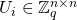to the matrices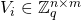.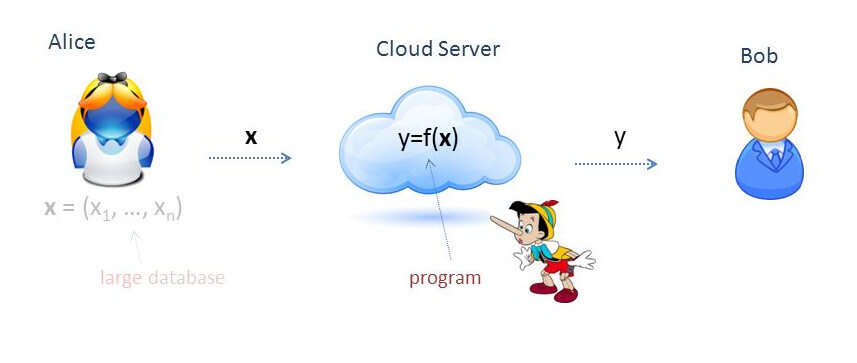Notice how the very idea of homomorphic signatures challenges the basic security requirements of traditional digital signatures. In fact, for a traditional signatures scheme we require that it should be computationally infeasible to generate a valid signature for a party without knowing that party’s private key. Here, we need to be able to generate a valid signature on some data (i.e. results of computation, like) without knowing the secret key. What we require, though, is that it must be computationally infeasible to forge a valid signature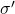for a result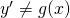. In other words, the security requirement is that it must not be possible to cheat on the signature of the result: if the provided result is validly signed, then it must be the correct result.

The next ideas stem from the analysis of the signature scheme devised by Gorbunov, Vaikuntanathan and Wichs. It relies on the Short Integer Solution hard problem on lattices. The scheme presents several limitations and possible improvements, but it is also the first homomorphic signature scheme able to evaluate arbitrary arithmetic circuits over signed data.

## But WHY is the Lattices Bounded Distance Decoding Problem difficult?

This is taken from my Master Thesis on Homomorphic Signatures over Lattices.

### Introduction to lattices and the Bounded Distance Decoding Problem

A lattice is a discrete subgroup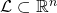, where the word discrete means that each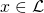has a neighborhood in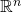that, when intersected with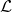results initself only. One can think of lattices as being grids, although the coordinates of the points need not be integer. Indeed, all lattices are isomorphic to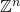, but it may be a grid of points with non-integer coordinates.Another very nice way to define a lattice is: givenindependent vectors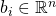, the latticegenerated by that base is the set of all linear combinations of them with integer coefficients: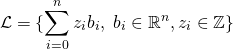Then, we can go on to define the Bounded Distance Decoding problem (BDD), which is used in lattice-based cryptography (more specifically, for example in trapdoor homomorphic encryption) and believed to be hard in general.

Given an arbitrary basis of a lattice, and a pointnot necessarily belonging to, find the point ofthat is closest to. We are also guaranteed thatis very close to one of the lattice points. Notice how we are relying on an arbitrary basis – if we claim to be able to solve the problem, we should be able to do so with any basis.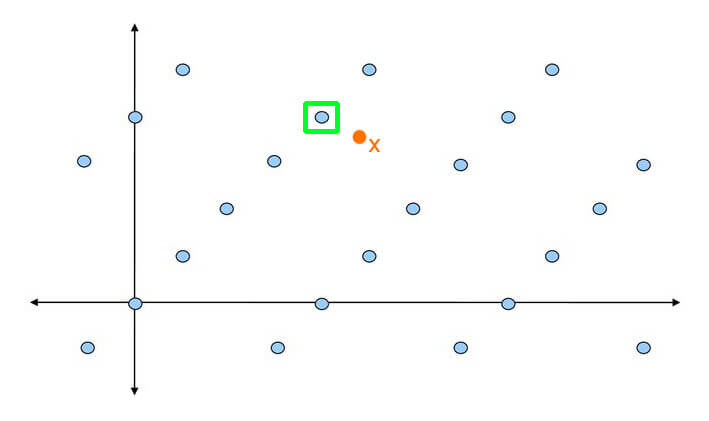Bounded Distance Problem example

Now, as the literature goes, this is a problem that is hard in general, but easy if the basis is nice enough. So, for example for encryption, the idea is that we can encode our secret message as a lattice point, and then add to it some small noise (i.e. a small element). This basically generates an instance of the BDD problem, and then the decoding can only be done by someone who holds the good basis for the lattice, while those having a bad basis are going to have a hard time decrypting the ciphertext.

However, albeit of course there is no proof of this (it is a problem believed to be hard), I wanted to get at least some clue on why it should be easy with a nice basis and hard with a bad one (GGH is an example schema that employs techniques based on this).

So now to our real question. But WHY is the Bounded Distance Decoding problem hard (or easy)?